Project showcase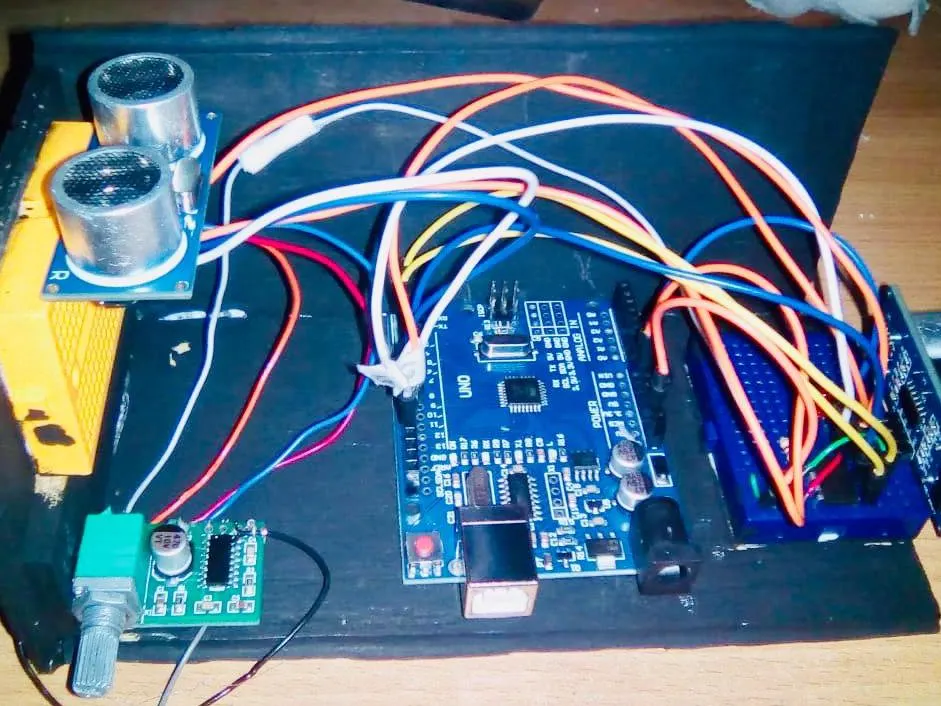A theremin made out of two ultrasonic sensors.

• 11,710 views
• 17 respects

Components and suppliesArduino UNO
×1Ultrasonic Sensor - HC-SR04 (Generic)
×2×3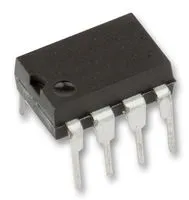Non Volatile Digital Potentiometer, 10 kohm we use a x9c103 model
×1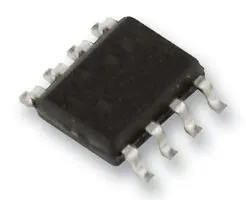Operational Amplifier, Op Amp + Comparator + Reference optional
×1Jumper wires (generic)
×1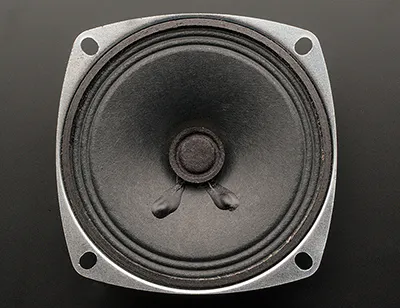Speaker: 3W, 4 ohms
×1LED (generic)
×1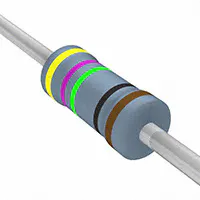Resistor 475 ohm
×1

Necessary tools and machinesHot glue gun (generic)

A theremin is an electronic musical instrument controlled without physical contact by the thereminist (performer). It was patented by Leon Theremin in 1928 and its controlling section usually consists of two metal antennas that sense the relative position of the thereminist's hands and control oscillators for frequency with one hand, and amplitude (volume) with the other. In the following project we try to emulate the functioning of this instrument using two ultrasonic sensors which are able to detected distances and as a function of this we tell the Arduino to emit sound at different frequencies and amplitudes through a loudspeaker. Here's a detailed explanation.

Frequency:

We use an ultrasonic sensor with four pins. Two are for voltage input and ground. Another one called trigger is used to send an ultrasonic pulse through a digital write on the program. The last pin is called echo and it recieves the ultrasonic pulse when it bounces back. The time it takes the pulse to go back to the board is read and stored in a variable, then we convert it to distance units (cm). Finally we use the TonePlayer library to convert each distance to a different frequency. We're using the equal temperament system to calculate exact musical notes, so for every different value of distance we have a different musical note.

Amplitude:

To control the amplitude we use another ultrasonic sensor but this time we decided to use the NewPing library and the sonar.ping_cm() function which sends a ping, returns the distance in centimeters or 0 (zero) if no ping echo within set distance limit. This information is stored in the variable distance. Then we made two special functions called incremento and decremento. This functions tell the digital potentiometer if it should increase or decrease the value of its resistance based on the distance information. If the distance is increased so is the resistance, if the distance is decreased then the resistance will decrease too.

Output:

A common loudspeaker is connected directly to the output pins of the digital potentiometer. The different values of resistance will be perceived as volume variations. Optionally we can use an amplifier for the speaker but it really isn’t necessary.

Remarks:

1) We used a common led as an indicator of the variation of resistance.

2) You gotta use the special TonePlayer library (link provided) cause any other similar library will crash Arduino whenever if runs with the NewPing library.

3) Tone functions only works on Arduino Uno. I'm not exactly sure why.

Here's the final result:

Code

Arduino ThereminArduino
Is a code for the arduino theremin
//In this project we create a theremin with two ultrasonic sensors, one for volume and another for tone. We use the digital potentiometer X9C103P.
/*
* Potentiometer conecctions:
* 1 - INC - Arduino pin 2
* 2 - U/D - Arduino pin 3
* 3 - VH  - 5V
* 4 - VSS - GND
* 5 - VW  - Output
* 6 - VL  - GND
* 7 - CS  - Arduino pin 4
* 8 - VCC - 5V
*
*/
//Note: we include two libraries: NewPing and TonePlayer because if we use the library NewPing with a tone function, the sketch doesnot compile.
#include <NewPing.h>
#include <TonePlayer.h>
#define MIN_DISTANCIA 35
TonePlayer tone1(TCCR1A, TCCR1B, OCR1AH, OCR1AL, TCNT1H, TCNT1L);

NewPing sonar(10, 11, 35); //The first and the second number are the pins of the sensor of volume, the third is the maximum distance

// name pins for sensors and the potentiometer
int echo = 12;
int trigger = 13;
int INC = 2;
int UD = 3;
int distancia;
int actual, anterior;
// necessary variables
unsigned long tiempoRespuesta;
float distancia2;
float tono1;

void setup() {
// set all the pins
Serial.begin(9600); //use of monitor serie for check the distance
pinMode(trigger, OUTPUT); //Tone sensor
pinMode(echo, INPUT); //Tone sensor
pinMode(2, OUTPUT); //Potentiometer
pinMode(3, OUTPUT); //Potentiometer
//pinMode (speaker, OUTPUT);

for(int j=0; j<90; j++){ //As we do not know in what value the potentiometer is, we place the value at the minimum
decremento();
}

}

void loop() {

digitalWrite(trigger, HIGH);
delayMicroseconds(10);   // Pulse of ten microseconds
digitalWrite(trigger, LOW);
tiempoRespuesta = pulseIn(echo, HIGH);  // Echo of the pulse
distancia2 = tiempoRespuesta/58;  //Calculation of the distance in centimeters

if (distancia2 < MIN_DISTANCIA) {
//Conversion of distance into a value for a sound
tono1 = 50.0*pow(2,(distancia2/12.0));  //pow alculates the value of a number raised to a power
pinMode(9, OUTPUT);
tone1.tone(tono1);  //Generates the tone with the previous value (220 Hz)
delay(10);
}

distancia = sonar.ping_cm(); //Calculation of the distance in centimeters (with the library NewPing)
Serial.print(distancia); //Check the distance
//Change the value "distancia", to values that are useful for the rise and fall of the potentiometer
actual = map(distancia, 0, 35, 0, 200);

//Compares the mapped values to raise or lower the potentiometer
if(actual > anterior){
for(int i = 0; i< (actual - anterior); i++){
decremento();
}
}

else{
for(int i = 0; i <= (anterior - actual); i++)
{
aumento();
}
}

anterior = actual; //The value is updated with each loop
delay(1);

}

//The U/D input controls the direction of the wiper movement and whether the counter is incremented or decremented
//The INC input is negative-edge triggered. Toggling INC will move the wiper and either increment or decrement the counter in the direction indicated by the logic level on the U/D input.
//The next two functions produce these toggling in the two directions (increment, decrement)

void aumento(){ //aumento == increment
digitalWrite(UD, HIGH);
digitalWrite(INC, LOW);
digitalWrite(INC, HIGH);
delay(10);
digitalWrite(INC, LOW);
}

void decremento(){ //decremento == decrement
digitalWrite(UD, LOW);
digitalWrite(INC, LOW);
digitalWrite(INC, HIGH);
delay(10);
digitalWrite(INC, LOW);
}

Schematics

Is an schematic for the Arduino theremin. Just copy the set up.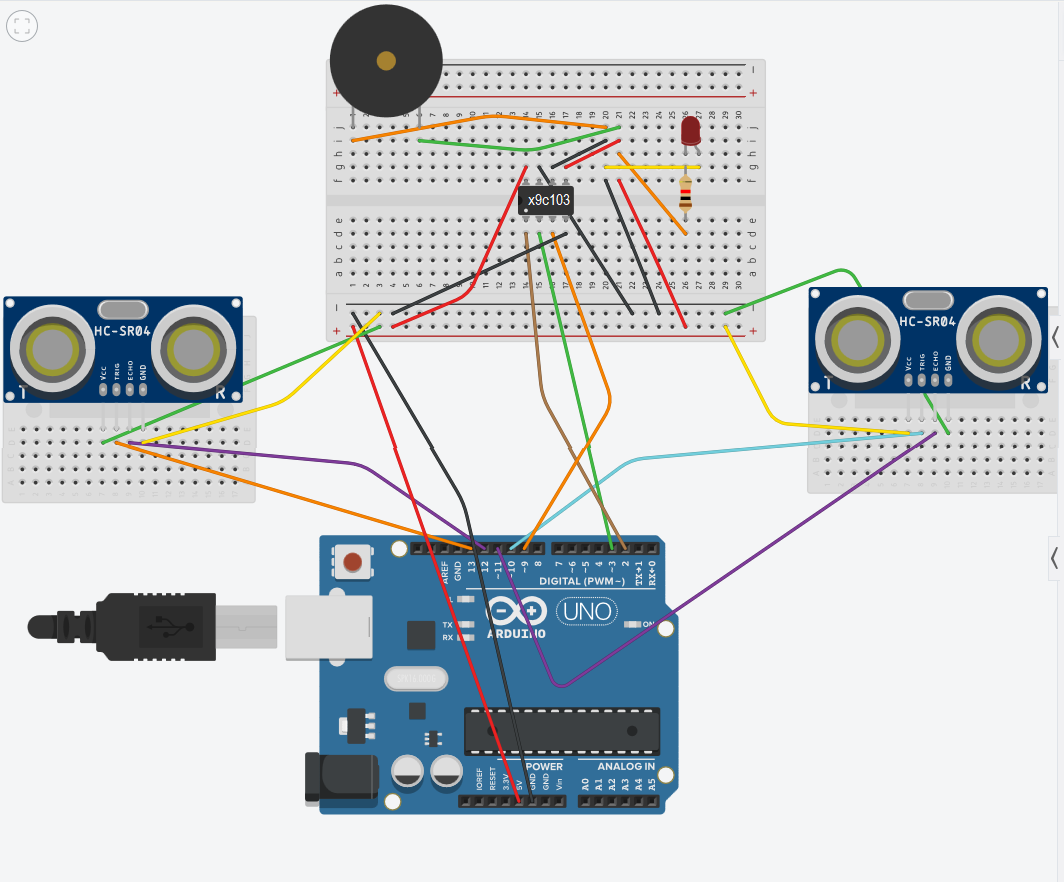Ultrasonic Theremin

Project showcase by jvmccall

• 4,378 views
• 6 respects

Theremino: a theremin made with Arduino

Project tutorial by tdelatorre and myfunnyandy

• 4,819 views
• 7 respects

Arduino Bluetooth Robot for Android Device

Project showcase by aip06

• 7,787 views
• 23 respects

Arduino Theremin with A Minor Pentatonic

Project tutorial by pollux labs

• 5,230 views
• 1 comment
• 16 respects

Electronic Piano Keyboard With Preset Songs

Project tutorial by Lindsay Fox

• 112,627 views